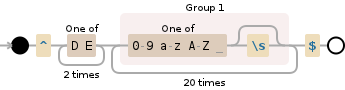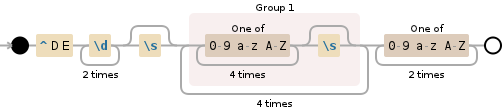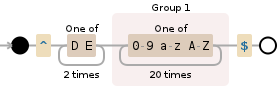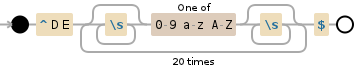# Regex for IBAN allowing for white spaces AND checking for exact length

I need to check an input field for a German IBAN. The user should be allowed to leave in white spaces and input should be validated to have a starting `DE` and then exact 20 characters numbers and letters.

Without the white space allowance, I tried

``````^[DE]{2}([0-9a-zA-Z]{20})\$
``````

but I cannot find where and how I can add "white spaces anywhere allowed.

This should be simple, but I simply cannot find a solution.

Thanks for help!

• What language are you using? Is removing all whitespaces from the string a suitable solution? Also, FYI, `[DE]{2}` matches `DD` or `ED`: see regular-expressions.info/charclass.html May 5, 2014 at 11:45
• as I'm saying in my answer, you're not using the right tool for the task. You should not check something as important as an IBAN against a format, but check the validity of the whole code by calculating the checksum, which is, agorithmically, as complex as a regex check.
– zmo
May 5, 2014 at 12:23
• Tanks a lot! we do validate the correct IBAN format properly in the backend, but want to guide the user frontend-wise already too. May 5, 2014 at 12:39
• do not tend to just guide, just validate it. To repeat myself: a checksum calcul is faster, lighter and easier to do than a regex, and it's the best way to validate an IBAN input!
– zmo
May 8, 2014 at 17:34

Because you should use the right tool for the right task: you should not rely on regexps to validate IBAN numbers, but instead use the IBAN checksum algorithm to check the whole code is actually correct, making any regexp superfluous and redundant. i.e.: remove all spaces, rearrange the code, convert to integers, and compute remainder, here it's best explained.

Though, there am I trying to answer your question, for the fun of it:

``````^DE([0-9a-zA-Z]\s?){20}\$
``````

which only difference is allowing a whitespace (or not) after each occurence of a alphanumeric character.

here is the visualization:edit: for the OP's information, the only difference is that this regexp, from @ulugbex-umirov: `(?:\s*[0-9a-zA-Z]\s*)` does a lookahead check to see if there's a space between the iso country code and the checksum (which only made of numerical digits), which I do not support on purpose.

And actually to support a correct IBAN syntax, which is formed of groups of 4 characters, as the wikipedia page says:

``````^DE\d{2}\s?([0-9a-zA-Z]{4}\s?){4}[0-9a-zA-Z]{2}\$
``````example

If your UI is in Javascript, you can use that library for doing IBAN validation:

``````<script src="iban.js"></script>
<script>
// the API is now accessible from the window.IBAN global object
IBAN.isValid('hello world'); // false
IBAN.isValid('BE68539007547034'); // true
</script>
``````

so you know this is a valid IBAN, and can validate it before the data is ever even sent to the backend. Simpler, lighter and more elegant… Why do something else?

• Good point about not relying on regexs to validate an IBAN, but I think a regex can be used in the frontend to catch any obvious mistakes and guide the user. I would do the more serious IBAN validation in the backend. May 5, 2014 at 12:38
• thanks a ton for you quick and very helpful answer! we do validate in the backend as well. the regex is additional, for user guidance May 5, 2014 at 12:40
• btw, if your UI is in javascript, there's a bunch of JS libraries: here, there or there that impements the checksum calculation.
– zmo
May 5, 2014 at 12:50
• @fbitterlich did you post detailed information about this bug anywhere? I don't see it as an issue on the arhs/iban.js github page. Jan 7, 2015 at 13:26
• @zmo: This answer makes me want to be able to upvote twice :-) Oct 21, 2015 at 6:58

Here is a list of IBANs from 70 Countries. I generated it with a python script i wrote based on this https://en.wikipedia.org/wiki/International_Bank_Account_Number

``````AL[a-zA-Z0-9]{2}\s?([0-9]{4}\s?){2}([a-zA-Z0-9]{4}\s?){4}\s?
AT[a-zA-Z0-9]{2}\s?([0-9]{4}\s?){4}\s?
AZ[a-zA-Z0-9]{2}\s?([a-zA-Z0-9]{4}\s?){1}([0-9]{4}\s?){5}\s?
BH[a-zA-Z0-9]{2}\s?([a-zA-Z]{4}\s?){1}([a-zA-Z0-9]{4}\s?){3}([a-zA-Z0-9]{2})\s?
BY[a-zA-Z0-9]{2}\s?([a-zA-Z0-9]{4}\s?){1}([0-9]{4}\s?){5}\s?
BE[a-zA-Z0-9]{2}\s?([0-9]{4}\s?){3}\s?
BA[a-zA-Z0-9]{2}\s?([0-9]{4}\s?){4}\s?
BR[a-zA-Z0-9]{2}\s?([0-9]{4}\s?){5}([0-9]{3})([a-zA-Z]{1}\s?)([a-zA-Z0-9]{1})\s?
BG[a-zA-Z0-9]{2}\s?([a-zA-Z]{4}\s?){1}([0-9]{4}\s?){1}([0-9]{2})([a-zA-Z0-9]{2}\s?)([a-zA-Z0-9]{4}\s?){1}([a-zA-Z0-9]{2})\s?
CR[a-zA-Z0-9]{2}\s?([0-9]{4}\s?){4}([0-9]{2})\s?
HR[a-zA-Z0-9]{2}\s?([0-9]{4}\s?){4}([0-9]{1})\s?
CY[a-zA-Z0-9]{2}\s?([0-9]{4}\s?){2}([a-zA-Z0-9]{4}\s?){4}\s?
CZ[a-zA-Z0-9]{2}\s?([0-9]{4}\s?){5}\s?
DK[a-zA-Z0-9]{2}\s?([0-9]{4}\s?){3}([0-9]{2})\s?
DO[a-zA-Z0-9]{2}\s?([a-zA-Z]{4}\s?){1}([0-9]{4}\s?){5}\s?
TL[a-zA-Z0-9]{2}\s?([0-9]{4}\s?){4}([0-9]{3})\s?
EE[a-zA-Z0-9]{2}\s?([0-9]{4}\s?){4}\s?
FO[a-zA-Z0-9]{2}\s?([0-9]{4}\s?){3}([0-9]{2})\s?
FI[a-zA-Z0-9]{2}\s?([0-9]{4}\s?){3}([0-9]{2})\s?
FR[a-zA-Z0-9]{2}\s?([0-9]{4}\s?){2}([0-9]{2})([a-zA-Z0-9]{2}\s?)([a-zA-Z0-9]{4}\s?){2}([a-zA-Z0-9]{1})([0-9]{2})\s?
GE[a-zA-Z0-9]{2}\s?([a-zA-Z0-9]{2})([0-9]{2}\s?)([0-9]{4}\s?){3}([0-9]{2})\s?
DE[a-zA-Z0-9]{2}\s?([0-9]{4}\s?){4}([0-9]{2})\s?
GI[a-zA-Z0-9]{2}\s?([a-zA-Z]{4}\s?){1}([a-zA-Z0-9]{4}\s?){3}([a-zA-Z0-9]{3})\s?
GR[a-zA-Z0-9]{2}\s?([0-9]{4}\s?){1}([0-9]{3})([a-zA-Z0-9]{1}\s?)([a-zA-Z0-9]{4}\s?){3}([a-zA-Z0-9]{3})\s?
GL[a-zA-Z0-9]{2}\s?([0-9]{4}\s?){3}([0-9]{2})\s?
GT[a-zA-Z0-9]{2}\s?([a-zA-Z0-9]{4}\s?){1}([a-zA-Z0-9]{4}\s?){5}\s?
HU[a-zA-Z0-9]{2}\s?([0-9]{4}\s?){6}\s?
IS[a-zA-Z0-9]{2}\s?([0-9]{4}\s?){5}([0-9]{2})\s?
IE[a-zA-Z0-9]{2}\s?([a-zA-Z0-9]{4}\s?){1}([0-9]{4}\s?){3}([0-9]{2})\s?
IL[a-zA-Z0-9]{2}\s?([0-9]{4}\s?){4}([0-9]{3})\s?
IT[a-zA-Z0-9]{2}\s?([a-zA-Z]{1})([0-9]{3}\s?)([0-9]{4}\s?){1}([0-9]{3})([a-zA-Z0-9]{1}\s?)([a-zA-Z0-9]{4}\s?){2}([a-zA-Z0-9]{3})\s?
JO[a-zA-Z0-9]{2}\s?([a-zA-Z]{4}\s?){1}([0-9]{4}\s?){5}([0-9]{2})\s?
KZ[a-zA-Z0-9]{2}\s?([0-9]{4}\s?){3}([0-9]{1})([a-zA-Z0-9]{3}\s?)([a-zA-Z0-9]{4}\s?){2}([a-zA-Z0-9]{2})\s?
XK[a-zA-Z0-9]{2}\s?([0-9]{4}\s?){1}([0-9]{4}\s?){2}([0-9]{2})([0-9]{2}\s?)\s?
KW[a-zA-Z0-9]{2}\s?([a-zA-Z]{4}\s?){1}([a-zA-Z0-9]{4}\s?){5}([a-zA-Z0-9]{2})\s?
LV[a-zA-Z0-9]{2}\s?([a-zA-Z]{4}\s?){1}([a-zA-Z0-9]{4}\s?){3}([a-zA-Z0-9]{1})\s?
LB[a-zA-Z0-9]{2}\s?([0-9]{4}\s?){1}([a-zA-Z0-9]{4}\s?){5}\s?
LI[a-zA-Z0-9]{2}\s?([0-9]{4}\s?){1}([0-9]{1})([a-zA-Z0-9]{3}\s?)([a-zA-Z0-9]{4}\s?){2}([a-zA-Z0-9]{1})\s?
LT[a-zA-Z0-9]{2}\s?([0-9]{4}\s?){4}\s?
LU[a-zA-Z0-9]{2}\s?([0-9]{3})([a-zA-Z0-9]{1}\s?)([a-zA-Z0-9]{4}\s?){3}\s?
MK[a-zA-Z0-9]{2}\s?([0-9]{3})([a-zA-Z0-9]{1}\s?)([a-zA-Z0-9]{4}\s?){2}([a-zA-Z0-9]{1})([0-9]{2})\s?
MT[a-zA-Z0-9]{2}\s?([a-zA-Z]{4}\s?){1}([0-9]{4}\s?){1}([0-9]{1})([a-zA-Z0-9]{3}\s?)([a-zA-Z0-9]{4}\s?){3}([a-zA-Z0-9]{3})\s?
MR[a-zA-Z0-9]{2}\s?([0-9]{4}\s?){5}([0-9]{3})\s?
MU[a-zA-Z0-9]{2}\s?([a-zA-Z]{4}\s?){1}([0-9]{4}\s?){4}([0-9]{3})([a-zA-Z]{1}\s?)([a-zA-Z]{2})\s?
MC[a-zA-Z0-9]{2}\s?([0-9]{4}\s?){2}([0-9]{2})([a-zA-Z0-9]{2}\s?)([a-zA-Z0-9]{4}\s?){2}([a-zA-Z0-9]{1})([0-9]{2})\s?
MD[a-zA-Z0-9]{2}\s?([a-zA-Z0-9]{2})([a-zA-Z0-9]{2}\s?)([a-zA-Z0-9]{4}\s?){4}\s?
ME[a-zA-Z0-9]{2}\s?([0-9]{4}\s?){4}([0-9]{2})\s?
NL[a-zA-Z0-9]{2}\s?([a-zA-Z]{4}\s?){1}([0-9]{4}\s?){2}([0-9]{2})\s?
NO[a-zA-Z0-9]{2}\s?([0-9]{4}\s?){2}([0-9]{3})\s?
PK[a-zA-Z0-9]{2}\s?([a-zA-Z0-9]{4}\s?){1}([0-9]{4}\s?){4}\s?
PS[a-zA-Z0-9]{2}\s?([a-zA-Z0-9]{4}\s?){1}([0-9]{4}\s?){5}([0-9]{1})\s?
PL[a-zA-Z0-9]{2}\s?([0-9]{4}\s?){6}\s?
PT[a-zA-Z0-9]{2}\s?([0-9]{4}\s?){5}([0-9]{1})\s?
QA[a-zA-Z0-9]{2}\s?([a-zA-Z]{4}\s?){1}([a-zA-Z0-9]{4}\s?){5}([a-zA-Z0-9]{1})\s?
RO[a-zA-Z0-9]{2}\s?([a-zA-Z]{4}\s?){1}([a-zA-Z0-9]{4}\s?){4}\s?
SM[a-zA-Z0-9]{2}\s?([a-zA-Z]{1})([0-9]{3}\s?)([0-9]{4}\s?){1}([0-9]{3})([a-zA-Z0-9]{1}\s?)([a-zA-Z0-9]{4}\s?){2}([a-zA-Z0-9]{3})\s?
SA[a-zA-Z0-9]{2}\s?([0-9]{2})([a-zA-Z0-9]{2}\s?)([a-zA-Z0-9]{4}\s?){4}\s?
RS[a-zA-Z0-9]{2}\s?([0-9]{4}\s?){4}([0-9]{2})\s?
SK[a-zA-Z0-9]{2}\s?([0-9]{4}\s?){5}\s?
SI[a-zA-Z0-9]{2}\s?([0-9]{4}\s?){3}([0-9]{3})\s?
ES[a-zA-Z0-9]{2}\s?([0-9]{4}\s?){5}\s?
SE[a-zA-Z0-9]{2}\s?([0-9]{4}\s?){5}\s?
CH[a-zA-Z0-9]{2}\s?([0-9]{4}\s?){1}([0-9]{1})([a-zA-Z0-9]{3}\s?)([a-zA-Z0-9]{4}\s?){2}([a-zA-Z0-9]{1})\s?
TN[a-zA-Z0-9]{2}\s?([0-9]{4}\s?){5}\s?
TR[a-zA-Z0-9]{2}\s?([0-9]{4}\s?){1}([0-9]{1})([a-zA-Z0-9]{3}\s?)([a-zA-Z0-9]{4}\s?){3}([a-zA-Z0-9]{2})\s?
AE[a-zA-Z0-9]{2}\s?([0-9]{3})([0-9]{1}\s?)([0-9]{4}\s?){3}([0-9]{3})\s?
GB[a-zA-Z0-9]{2}\s?([a-zA-Z]{4}\s?){1}([0-9]{4}\s?){3}([0-9]{2})\s?
VA[a-zA-Z0-9]{2}\s?([0-9]{3})([0-9]{1}\s?)([0-9]{4}\s?){3}([0-9]{2})\s?
VG[a-zA-Z0-9]{2}\s?([a-zA-Z0-9]{4}\s?){1}([0-9]{4}\s?){4}\s?
``````
• Don't know why the downvotes. This list is at least incredibly helpful. These seem correct. And the German regex is in your answer. Oct 25, 2019 at 12:59

Original:

``````^[DE]{2}([0-9a-zA-Z]{20})\$
``````Debuggex Demo

Modified:

``````^DE(?:\s*[0-9a-zA-Z]\s*){20}\$
``````Debuggex Demo

This is the correct regex to match DE IBAN account numbers:

``````DE\d{2}[ ]\d{4}[ ]\d{4}[ ]\d{4}[ ]\d{4}[ ]\d{2}|DE\d{20}

Pass:   DE89 3704 0044 0532 0130 00|||DE89370400440532013000
Fail:   DE89-3704-0044-0532-0130-00
``````

Most simple solution I can think of:

``````^DE(\s*[[:alnum:]]){20}\s*\$
``````

In particular, your initial `[DE]{2}` is wrong, as it allows 'DD', 'EE', 'ED' as well as the intended 'DE'.

To allow any amount of spaces anywhere:

``````^ *D *E( *[A-Za-z0-9]){20} *\$
``````

As you want to allow lower letters, also `DE` might be lower?

``````^ *[Dd] *[Ee]( *[A-Za-z0-9]){20} *\$
``````
• `^` matches the start of the string
• `\$` end anchor
• in between each characters there are optional spaces `*`
• `[`character class`]` defines a set/range of characters

To allow at most one space in between each characters, replace the quantifier `*` (any amount of) with `?` (0 or 1). If supported, `\s` shorthand can be used to match `[ \t\r\n\f]` instead of space only.

Test on regex101.com, also see the SO regex FAQ

Using Google Apps Script, I pasted Laurent's code from github into a script and added the following code to test.

``````// Use the Apps Script IDE's "Run" menu to execute this code.
// Then look at the View > Logs menu to see execution results.

function myFunction() {
The only thing needed to run the example code was commenting out the `require('iban')` line: // var IBAN = require('iban'); Finally, instead of using client handlers to attempt a RegEx validation of IBAN input, I use a a server handler to do the validation.